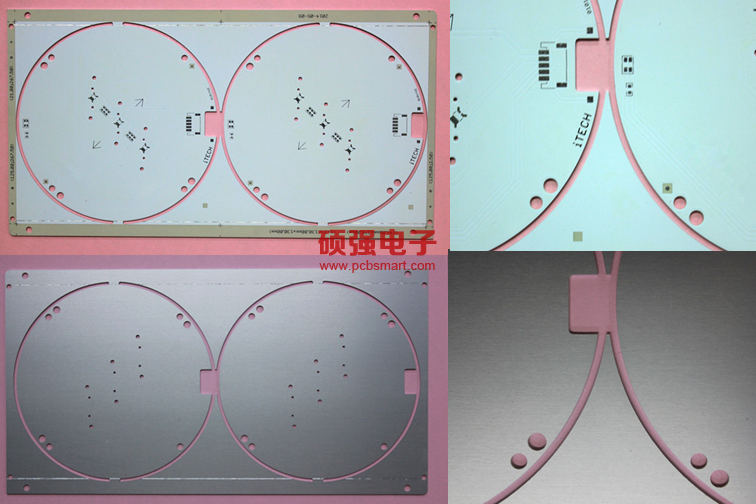1um=？ mm,1mil=？ Conversion formula of PCB unit in mm circuit board

People engaged in PCB industry will inevitably encounter all kinds of data units. The conversion between various units is often used. It is often necessary to check and find out the conversion value. In order to facilitate the use and learning of PCB practitioners, PCB engineering, PCB electronic engineers and other personnel. The following are examples of conversion values between various units for your reference. Both Chinese and English abbreviations are noted. I believe that the following unit conversion, is the value you keep learning. The following examples of conversion values between various units for your reference. Both Chinese and English abbreviations are noted. I believe that the following unit conversion is worth your learningCommon unit of line width / line distance / plating thickness: 1um (1 μ m) = 0.001mm (0.001mm)

1um=0.03937 mil

1um = 40uin

1um (1 μ m) = 0.001mm

1mil = 0.0254mm (conversion source: 1mil = 1 / 1000inch = 0.00254cm = 0.0254mm)

It is known that: 4mil / 4mil = 0.1mm/0.1mm linewidth: 1mil = 25.4um, 1mil = 1000uin mil. Sometimes, it can also be divided into British wire two (commonly used unit of PCB copper thickness) 1oz (ounces) = 35um (micrometer) = 28.35g/square foot

1oz (oz) = 28.35g, which is the British unit

1oz (oz) = 28.35g/square foot = 35um μ M H / h = 18um / 18um micron double-sided plate, both sides are 18um copper thickness, three relative not commonly used bit conversion:

1 foot = 12 inches

1 inch inch = 1000 mil

1asd = 1 ampere / square decimeter = 10.76 ampere / square foot (1 square decimeter = 10.76 square feet)

1am = 1 ampere min = 60 Coulomb, mainly used for precious metal electroplating, such as gold plating

1 square foot = 9.29 square decimeters 1 gallon (British system) = 4.5 liters

1 gallon us = 3.785 liters

1kha = 1000 ah

1 ampere hour = 3600 Coulomb